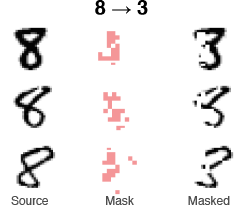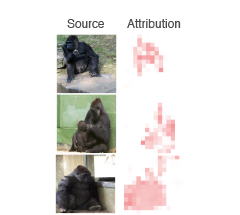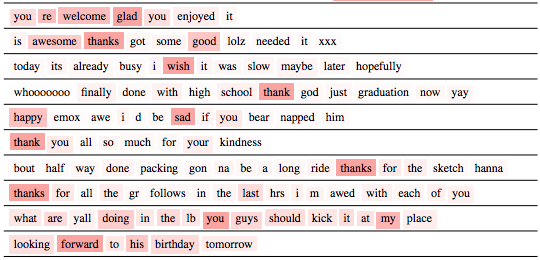Causal Explanations (CXPlain) is a method for explaining the decisions of any machine-learning model. CXPlain uses explanation models trained with a causal objective to learn to explain machine-learning models, and to quantify the uncertainty of its explanations. This repository contains a reference implementation for neural explanation models, and several practical examples for different data modalities. Please see the manuscript at https://arxiv.org/abs/1910.12336 (NeurIPS 2019) for a description and experimental evaluation of CXPlain.

## Install

To install the latest release:

``\$ pip install cxplain``

## Use

A CXPlain model consists of four main components:

• The model to be explained which can be any type of machine-learning model, including black-box models, such as neural networks and ensemble models.
• The model builder that defines the structure of the explanation model to be used to explain the explained model.
• The masking operation that defines how CXPlain will internally simulate the removal of input features from the set of available features.
• The loss function that defines how the change in prediction accuracy incurred by removing an input feature will be measured by CXPlain.

After configuring these four components, you can fit a CXPlain instance to the same training data that was used to train your original model. The CXPlain instance can then explain any prediction of your explained model - even when no labels are available for that sample.

``````from tensorflow.python.keras.losses import categorical_crossentropy
from cxplain import MLPModelBuilder, ZeroMasking, CXPlain

x_train, y_train, x_test = ....  # Your dataset
explained_model = ...    # The model you wish to explain.

# Define the model you want to use to explain your __explained_model__.
# Here, we use a neural explanation model with a
# multilayer perceptron (MLP) architecture.
model_builder = MLPModelBuilder(num_layers=2, num_units=64, batch_size=256, learning_rate=0.001)

# removal of input features used internally by CXPlain - ZeroMasking is typically a sensible default choice for tabular and image data.

# Define the loss with which each input features' associated reduction in prediction error is calculated.
loss = categorical_crossentropy

# Build and fit a CXPlain instance.
explainer = CXPlain(explained_model, model_builder, masking_operation, loss)
explainer.fit(x_train, y_train)

# Use the __explainer__ to obtain explanations for the predictions of your __explained_model__.

## Examples

More practical examples for various input data modalities, including images, textual data and tabular data, and both regression and classification tasks are provided in form of Jupyter notebooks in the examples/ directory:## Cite

Please consider citing, if you reference or use our methodology, code or results in your work:

``````@inproceedings{schwab2019cxplain,
title={{CXPlain: Causal Explanations for Model Interpretation under Uncertainty}},
author={Schwab, Patrick and Karlen, Walter},
booktitle={{Advances in Neural Information Processing Systems (NeurIPS)}},
year={2019}
}``````